Linear Regression and Correlation

# 71 The Regression Equation

Regression analysis is a statistical technique that can test the hypothesis that a variable is dependent upon one or more other variables. Further, regression analysis can provide an estimate of the magnitude of the impact of a change in one variable on another. This last feature, of course, is all important in predicting future values.

Regression analysis is based upon a functional relationship among variables and further, assumes that the relationship is linear. This linearity assumption is required because, for the most part, the theoretical statistical properties of non-linear estimation are not well worked out yet by the mathematicians and econometricians. This presents us with some difficulties in economic analysis because many of our theoretical models are nonlinear. The marginal cost curve, for example, is decidedly nonlinear as is the total cost function, if we are to believe in the effect of specialization of labor and the Law of Diminishing Marginal Product. There are techniques for overcoming some of these difficulties, exponential and logarithmic transformation of the data for example, but at the outset we must recognize that standard ordinary least squares (OLS) regression analysis will always use a linear function to estimate what might be a nonlinear relationship.

The general linear regression model can be stated by the equation: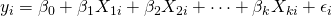where β0 is the intercept, βi‘s are the slope between Y and the appropriate Xi, and ε (pronounced epsilon), is the error term that captures errors in measurement of Y and the effect on Y of any variables missing from the equation that would contribute to explaining variations in Y. This equation is the theoretical population equation and therefore uses Greek letters. The equation we will estimate will have the Roman equivalent symbols. This is parallel to how we kept track of the population parameters and sample parameters before. The symbol for the population mean was µ and for the sample mean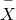and for the population standard deviation was σ and for the sample standard deviation was s. The equation that will be estimated with a sample of data for two independent variables will thus be: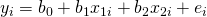As with our earlier work with probability distributions, this model works only if certain assumptions hold. These are that the Y is normally distributed, the errors are also normally distributed with a mean of zero and a constant standard deviation, and that the error terms are independent of the size of X and independent of each other.

### Assumptions of the Ordinary Least Squares Regression Model

Each of these assumptions needs a bit more explanation. If one of these assumptions fails to be true, then it will have an effect on the quality of the estimates. Some of the failures of these assumptions can be fixed while others result in estimates that quite simply provide no insight into the questions the model is trying to answer or worse, give biased estimates.

1. The independent variables,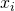, are all measured without error, and are fixed numbers that are independent of the error term. This assumption is saying in effect that Y is deterministic, the result of a fixed component “X” and a random error component “ϵ.”
2. The error term is a random variable with a mean of zero and a constant variance. The meaning of this is that the variances of the independent variables are independent of the value of the variable. Consider the relationship between personal income and the quantity of a good purchased as an example of a case where the variance is dependent upon the value of the independent variable, income. It is plausible that as income increases the variation around the amount purchased will also increase simply because of the flexibility provided with higher levels of income. The assumption is for constant variance with respect to the magnitude of the independent variable called homoscedasticity. If the assumption fails, then it is called heteroscedasticity. (Figure) shows the case of homoscedasticity where all three distributions have the same variance around the predicted value of Y regardless of the magnitude of X.
3. While the independent variables are all fixed values they are from a probability distribution that is normally distributed. This can be seen in (Figure) by the shape of the distributions placed on the predicted line at the expected value of the relevant value of Y.
4. The independent variables are independent of Y, but are also assumed to be independent of the other X variables. The model is designed to estimate the effects of independent variables on some dependent variable in accordance with a proposed theory. The case where some or more of the independent variables are correlated is not unusual. There may be no cause and effect relationship among the independent variables, but nevertheless they move together. Take the case of a simple supply curve where quantity supplied is theoretically related to the price of the product and the prices of inputs. There may be multiple inputs that may over time move together from general inflationary pressure. The input prices will therefore violate this assumption of regression analysis. This condition is called multicollinearity, which will be taken up in detail later.
5. The error terms are uncorrelated with each other. This situation arises from an effect on one error term from another error term. While not exclusively a time series problem, it is here that we most often see this case. An X variable in time period one has an effect on the Y variable, but this effect then has an effect in the next time period. This effect gives rise to a relationship among the error terms. This case is called autocorrelation, “self-correlated.” The error terms are now not independent of each other, but rather have their own effect on subsequent error terms.

(Figure) shows the case where the assumptions of the regression model are being satisfied. The estimated line is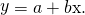Three values of X are shown. A normal distribution is placed at each point where X equals the estimated line and the associated error at each value of Y. Notice that the three distributions are normally distributed around the point on the line, and further, the variation, variance, around the predicted value is constant indicating homoscedasticity from assumption 2. (Figure) does not show all the assumptions of the regression model, but it helps visualize these important ones.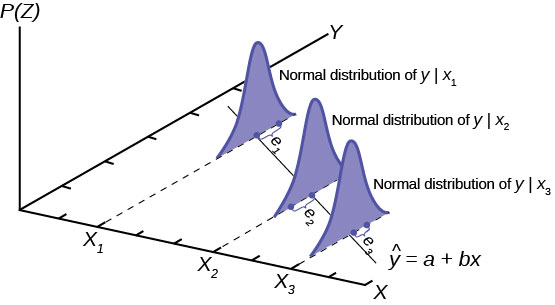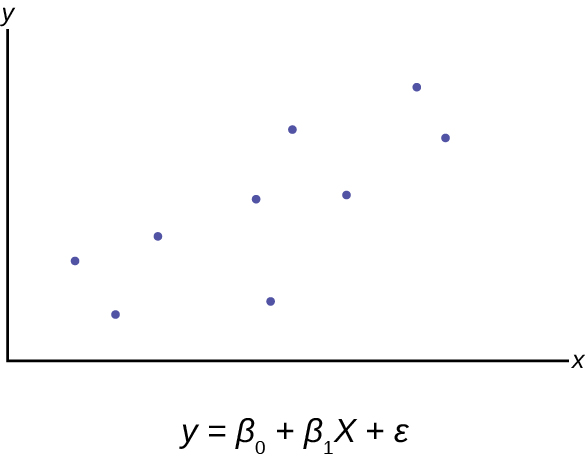This is the general form that is most often called the multiple regression model. So-called “simple” regression analysis has only one independent (right-hand) variable rather than many independent variables. Simple regression is just a special case of multiple regression. There is some value in beginning with simple regression: it is easy to graph in two dimensions, difficult to graph in three dimensions, and impossible to graph in more than three dimensions. Consequently, our graphs will be for the simple regression case. (Figure) presents the regression problem in the form of a scatter plot graph of the data set where it is hypothesized that Y is dependent upon the single independent variable X.

A basic relationship from Macroeconomic Principles is the consumption function. This theoretical relationship states that as a person’s income rises, their consumption rises, but by a smaller amount than the rise in income. If Y is consumption and X is income in the equation below (Figure), the regression problem is, first, to establish that this relationship exists, and second, to determine the impact of a change in income on a person’s consumption. The parameter β1 was called the Marginal Propensity to Consume in Macroeconomics Principles.

Each “dot” in (Figure) represents the consumption and income of different individuals at some point in time. This was called cross-section data earlier; observations on variables at one point in time across different people or other units of measurement. This analysis is often done with time series data, which would be the consumption and income of one individual or country at different points in time. For macroeconomic problems it is common to use times series aggregated data for a whole country. For this particular theoretical concept these data are readily available in the annual report of the President’s Council of Economic Advisors.

The regression problem comes down to determining which straight line would best represent the data in (Figure). Regression analysis is sometimes called “least squares” analysis because the method of determining which line best “fits” the data is to minimize the sum of the squared residuals of a line put through the data.

Population Equation: C = β0 + β1 Income + ε
Estimated Equation: C = b0 + b1 Income + eThis figure shows the assumed relationship between consumption and income from macroeconomic theory. Here the data are plotted as a scatter plot and an estimated straight line has been drawn. From this graph we can see an error term, e1. Each data point also has an error term. Again, the error term is put into the equation to capture effects on consumption that are not caused by income changes. Such other effects might be a person’s savings or wealth, or periods of unemployment. We will see how by minimizing the sum of these errors we can get an estimate for the slope and intercept of this line.

Consider the graph below. The notation has returned to that for the more general model rather than the specific case of the Macroeconomic consumption function in our example.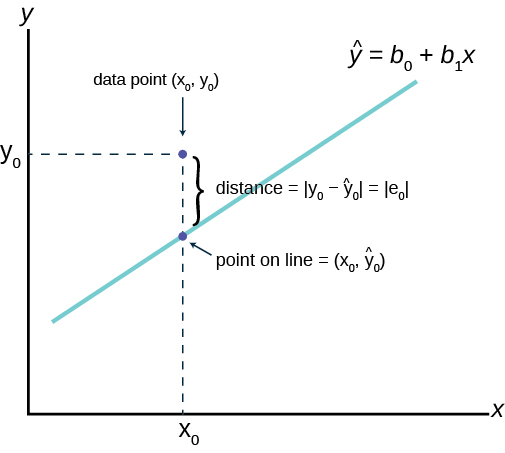The ŷ is read y hat” and is the estimated value of y. (In (Figure)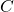represents the estimated value of consumption because it is on the estimated line.) It is the value of y obtained using the regression line. ŷ is not generally equal to y from the data.

The term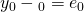is called the “error” or residual. It is not an error in the sense of a mistake. The error term was put into the estimating equation to capture missing variables and errors in measurement that may have occurred in the dependent variables. The absolute value of a residual measures the vertical distance between the actual value of y and the estimated value of y. In other words, it measures the vertical distance between the actual data point and the predicted point on the line as can be seen on the graph at point X0.

If the observed data point lies above the line, the residual is positive, and the line underestimates the actual data value for y.

If the observed data point lies below the line, the residual is negative, and the line overestimates that actual data value for y.

In the graph,is the residual for the point shown. Here the point lies above the line and the residual is positive. For each data point the residuals, or errors, are calculated yi – ŷi = ei for i = 1, 2, 3, …, n where n is the sample size. Each |e| is a vertical distance.

The sum of the errors squared is the term obviously called Sum of Squared Errors (SSE).

Using calculus, you can determine the straight line that has the parameter values of b0 and b1 that minimizes the SSE. When you make the SSE a minimum, you have determined the points that are on the line of best fit. It turns out that the line of best fit has the equation: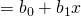where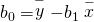and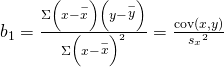The sample means of the x values and the y values are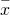and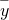, respectively. The best fit line always passes through the point (,) called the points of means.

The slope b can also be written as: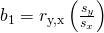where sy = the standard deviation of the y values and sx = the standard deviation of the x values and r is the correlation coefficient between x and y.

These equations are called the Normal Equations and come from another very important mathematical finding called the Gauss-Markov Theorem without which we could not do regression analysis. The Gauss-Markov Theorem tells us that the estimates we get from using the ordinary least squares (OLS) regression method will result in estimates that have some very important properties. In the Gauss-Markov Theorem it was proved that a least squares line is BLUE, which is, Best, Linear, Unbiased, Estimator. Best is the statistical property that an estimator is the one with the minimum variance. Linear refers to the property of the type of line being estimated. An unbiased estimator is one whose estimating function has an expected mean equal to the mean of the population. (You will remember that the expected value of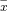was equal to the population mean µ in accordance with the Central Limit Theorem. This is exactly the same concept here).

Both Gauss and Markov were giants in the field of mathematics, and Gauss in physics too, in the 18th century and early 19th century. They barely overlapped chronologically and never in geography, but Markov’s work on this theorem was based extensively on the earlier work of Carl Gauss. The extensive applied value of this theorem had to wait until the middle of this last century.

Using the OLS method we can now find the estimate of the error variance which is the variance of the squared errors, e2. This is sometimes called the standard error of the estimate. (Grammatically this is probably best said as the estimate of the error’s variance) The formula for the estimate of the error variance is: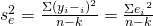where ŷ is the predicted value of y and y is the observed value, and thus the term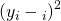is the squared errors that are to be minimized to find the estimates of the regression line parameters. This is really just the variance of the error terms and follows our regular variance formula. One important note is that here we are dividing by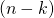, which is the degrees of freedom. The degrees of freedom of a regression equation will be the number of observations, n, reduced by the number of estimated parameters, which includes the intercept as a parameter.

The variance of the errors is fundamental in testing hypotheses for a regression. It tells us just how “tight” the dispersion is about the line. As we will see shortly, the greater the dispersion about the line, meaning the larger the variance of the errors, the less probable that the hypothesized independent variable will be found to have a significant effect on the dependent variable. In short, the theory being tested will more likely fail if the variance of the error term is high. Upon reflection this should not be a surprise. As we tested hypotheses about a mean we observed that large variances reduced the calculated test statistic and thus it failed to reach the tail of the distribution. In those cases, the null hypotheses could not be rejected. If we cannot reject the null hypothesis in a regression problem, we must conclude that the hypothesized independent variable has no effect on the dependent variable.

A way to visualize this concept is to draw two scatter plots of x and y data along a predetermined line. The first will have little variance of the errors, meaning that all the data points will move close to the line. Now do the same except the data points will have a large estimate of the error variance, meaning that the data points are scattered widely along the line. Clearly the confidence about a relationship between x and y is effected by this difference between the estimate of the error variance.

### Testing the Parameters of the Line

The whole goal of the regression analysis was to test the hypothesis that the dependent variable, Y, was in fact dependent upon the values of the independent variables as asserted by some foundation theory, such as the consumption function example. Looking at the estimated equation under (Figure), we see that this amounts to determining the values of b0 and b1. Notice that again we are using the convention of Greek letters for the population parameters and Roman letters for their estimates.

The regression analysis output provided by the computer software will produce an estimate of b0 and b1, and any other b’s for other independent variables that were included in the estimated equation. The issue is how good are these estimates? In order to test a hypothesis concerning any estimate, we have found that we need to know the underlying sampling distribution. It should come as no surprise at his stage in the course that the answer is going to be the normal distribution. This can be seen by remembering the assumption that the error term in the population, ε, is normally distributed. If the error term is normally distributed and the variance of the estimates of the equation parameters, b0 and b1, are determined by the variance of the error term, it follows that the variances of the parameter estimates are also normally distributed. And indeed this is just the case.

We can see this by the creation of the test statistic for the test of hypothesis for the slope parameter, β1 in our consumption function equation. To test whether or not Y does indeed depend upon X, or in our example, that consumption depends upon income, we need only test the hypothesis that β1 equals zero. This hypothesis would be stated formally as: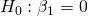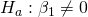If we cannot reject the null hypothesis, we must conclude that our theory has no validity. If we cannot reject the null hypothesis that β1 = 0 then b1, the coefficient of Income, is zero and zero times anything is zero. Therefore the effect of Income on Consumption is zero. There is no relationship as our theory had suggested.

Notice that we have set up the presumption, the null hypothesis, as “no relationship”. This puts the burden of proof on the alternative hypothesis. In other words, if we are to validate our claim of finding a relationship, we must do so with a level of significance greater than 90, 95, or 99 percent. The status quo is ignorance, no relationship exists, and to be able to make the claim that we have actually added to our body of knowledge we must do so with significant probability of being correct. John Maynard Keynes got it right and thus was born Keynesian economics starting with this basic concept in 1936.

The test statistic for this test comes directly from our old friend the standardizing formula: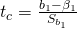where b1 is the estimated value of the slope of the regression line, β1 is the hypothesized value of beta, in this case zero, and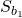is the standard deviation of the estimate of b1. In this case we are asking how many standard deviations is the estimated slope away from the hypothesized slope. This is exactly the same question we asked before with respect to a hypothesis about a mean: how many standard deviations is the estimated mean, the sample mean, from the hypothesized mean?

The test statistic is written as a student’s t distribution, but if the sample size is larger enough so that the degrees of freedom are greater than 30 we may again use the normal distribution. To see why we can use the student’s t or normal distribution we have only to look at,the formula for the standard deviation of the estimate of b1: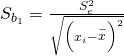or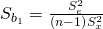Where Se is the estimate of the error variance and S2x is the variance of x values of the coefficient of the independent variable being tested.

We see that Se, the estimate of the error variance, is part of the computation. Because the estimate of the error variance is based on the assumption of normality of the error terms, we can conclude that the sampling distribution of the b’s, the coefficients of our hypothesized regression line, are also normally distributed.

One last note concerns the degrees of freedom of the test statistic, ν=n-k. Previously we subtracted 1 from the sample size to determine the degrees of freedom in a student’s t problem. Here we must subtract one degree of freedom for each parameter estimated in the equation. For the example of the consumption function we lose 2 degrees of freedom, one for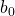, the intercept, and one for b1, the slope of the consumption function. The degrees of freedom would be n – k – 1, where k is the number of independent variables and the extra one is lost because of the intercept. If we were estimating an equation with three independent variables, we would lose 4 degrees of freedom: three for the independent variables, k, and one more for the intercept.

The decision rule for acceptance or rejection of the null hypothesis follows exactly the same form as in all our previous test of hypothesis. Namely, if the calculated value of t (or Z) falls into the tails of the distribution, where the tails are defined by α ,the required significance level in the test, we cannot accept the null hypothesis. If on the other hand, the calculated value of the test statistic is within the critical region, we cannot reject the null hypothesis.

If we conclude that we cannot accept the null hypothesis, we are able to state with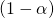level of confidence that the slope of the line is given by b1. This is an extremely important conclusion. Regression analysis not only allows us to test if a cause and effect relationship exists, we can also determine the magnitude of that relationship, if one is found to exist. It is this feature of regression analysis that makes it so valuable. If models can be developed that have statistical validity, we are then able to simulate the effects of changes in variables that may be under our control with some degree of probability , of course. For example, if advertising is demonstrated to effect sales, we can determine the effects of changing the advertising budget and decide if the increased sales are worth the added expense.

### Multicollinearity

Our discussion earlier indicated that like all statistical models, the OLS regression model has important assumptions attached. Each assumption, if violated, has an effect on the ability of the model to provide useful and meaningful estimates. The Gauss-Markov Theorem has assured us that the OLS estimates are unbiased and minimum variance, but this is true only under the assumptions of the model. Here we will look at the effects on OLS estimates if the independent variables are correlated. The other assumptions and the methods to mitigate the difficulties they pose if they are found to be violated are examined in Econometrics courses. We take up multicollinearity because it is so often prevalent in Economic models and it often leads to frustrating results.

The OLS model assumes that all the independent variables are independent of each other. This assumption is easy to test for a particular sample of data with simple correlation coefficients. Correlation, like much in statistics, is a matter of degree: a little is not good, and a lot is terrible.

The goal of the regression technique is to tease out the independent impacts of each of a set of independent variables on some hypothesized dependent variable. If two 2 independent variables are interrelated, that is, correlated, then we cannot isolate the effects on Y of one from the other. In an extreme case where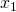is a linear combination of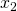, correlation equal to one, both variables move in identical ways with Y. In this case it is impossible to determine the variable that is the true cause of the effect on Y. (If the two variables were actually perfectly correlated, then mathematically no regression results could actually be calculated.)

The normal equations for the coefficients show the effects of multicollinearity on the coefficients.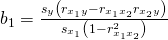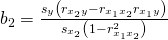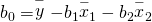The correlation betweenand,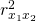, appears in the denominator of both the estimating formula for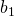and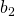. If the assumption of independence holds, then this term is zero. This indicates that there is no effect of the correlation on the coefficient. On the other hand, as the correlation between the two independent variables increases the denominator decreases, and thus the estimate of the coefficient increases. The correlation has the same effect on both of the coefficients of these two variables. In essence, each variable is “taking” part of the effect on Y that should be attributed to the collinear variable. This results in biased estimates.

Multicollinearity has a further deleterious impact on the OLS estimates. The correlation between the two independent variables also shows up in the formulas for the estimate of the variance for the coefficients.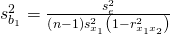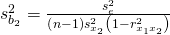Here again we see the correlation betweenandin the denominator of the estimates of the variance for the coefficients for both variables. If the correlation is zero as assumed in the regression model, then the formula collapses to the familiar ratio of the variance of the errors to the variance of the relevant independent variable. If however the two independent variables are correlated, then the variance of the estimate of the coefficient increases. This results in a smaller t-value for the test of hypothesis of the coefficient. In short, multicollinearity results in failing to reject the null hypothesis that the X variable has no impact on Y when in fact X does have a statistically significant impact on Y. Said another way, the large standard errors of the estimated coefficient created by multicollinearity suggest statistical insignificance even when the hypothesized relationship is strong.

### How Good is the Equation?

In the last section we concerned ourselves with testing the hypothesis that the dependent variable did indeed depend upon the hypothesized independent variable or variables. It may be that we find an independent variable that has some effect on the dependent variable, but it may not be the only one, and it may not even be the most important one. Remember that the error term was placed in the model to capture the effects of any missing independent variables. It follows that the error term may be used to give a measure of the “goodness of fit” of the equation taken as a whole in explaining the variation of the dependent variable, Y.

The multiple correlation coefficient, also called the coefficient of multiple determination or the coefficient of determination, is given by the formula: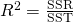where SSR is the regression sum of squares, the squared deviation of the predicted value of y from the mean value of y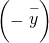, and SST is the total sum of squares which is the total squared deviation of the dependent variable, y, from its mean value, including the error term, SSE, the sum of squared errors. (Figure) shows how the total deviation of the dependent variable, y, is partitioned into these two pieces.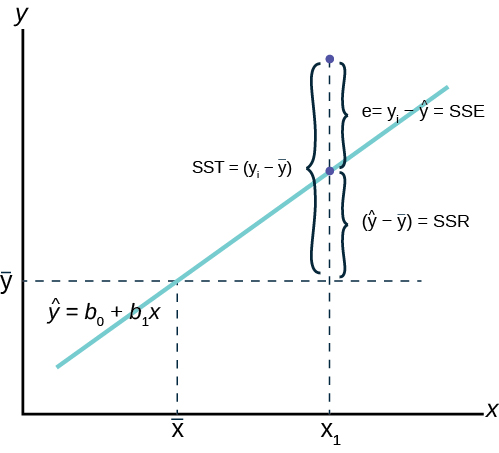(Figure) shows the estimated regression line and a single observation, x1. Regression analysis tries to explain the variation of the data about the mean value of the dependent variable, y. The question is, why do the observations of y vary from the average level of y? The value of y at observation x1 varies from the mean of y by the difference (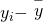). The sum of these differences squared is SST, the sum of squares total. The actual value of y at x1 deviates from the estimated value, ŷ, by the difference between the estimated value and the actual value, (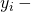). We recall that this is the error term, e, and the sum of these errors is SSE, sum of squared errors. The deviation of the predicted value of y, ŷ, from the mean value of y is (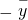) and is the SSR, sum of squares regression. It is called “regression” because it is the deviation explained by the regression. (Sometimes the SSR is called SSM for sum of squares mean because it measures the deviation from the mean value of the dependent variable, y, as shown on the graph.).

Because the SST = SSR + SSE we see that the multiple correlation coefficient is the percent of the variance, or deviation in y from its mean value, that is explained by the equation when taken as a whole. R2 will vary between zero and 1, with zero indicating that none of the variation in y was explained by the equation and a value of 1 indicating that 100% of the variation in y was explained by the equation. For time series studies expect a high R2 and for cross-section data expect low R2.

While a high R2 is desirable, remember that it is the tests of the hypothesis concerning the existence of a relationship between a set of independent variables and a particular dependent variable that was the motivating factor in using the regression model. It is validating a cause and effect relationship developed by some theory that is the true reason that we chose the regression analysis. Increasing the number of independent variables will have the effect of increasing R2. To account for this effect the proper measure of the coefficient of determination is the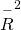, adjusted for degrees of freedom, to keep down mindless addition of independent variables.

There is no statistical test for the R2 and thus little can be said about the model using R2 with our characteristic confidence level. Two models that have the same size of SSE, that is sum of squared errors, may have very different R2 if the competing models have different SST, total sum of squared deviations. The goodness of fit of the two models is the same; they both have the same sum of squares unexplained, errors squared, but because of the larger total sum of squares on one of the models the R2 differs. Again, the real value of regression as a tool is to examine hypotheses developed from a model that predicts certain relationships among the variables. These are tests of hypotheses on the coefficients of the model and not a game of maximizing R2.

Another way to test the general quality of the overall model is to test the coefficients as a group rather than independently. Because this is multiple regression (more than one X), we use the F-test to determine if our coefficients collectively affect Y. The hypothesis is: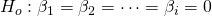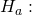“at least one of the βi is not equal to 0”

If the null hypothesis cannot be rejected, then we conclude that none of the independent variables contribute to explaining the variation in Y. Reviewing (Figure) we see that SSR, the explained sum of squares, is a measure of just how much of the variation in Y is explained by all the variables in the model. SSE, the sum of the errors squared, measures just how much is unexplained. It follows that the ratio of these two can provide us with a statistical test of the model as a whole. Remembering that the F distribution is a ratio of Chi squared distributions and that variances are distributed according to Chi Squared, and the sum of squared errors and the sum of squares are both variances, we have the test statistic for this hypothesis as: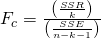where n is the number of observations and k is the number of independent variables. It can be shown that this is equivalent to: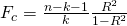(Figure) where R2 is the coefficient of determination which is also a measure of the “goodness” of the model.

As with all our tests of hypothesis, we reach a conclusion by comparing the calculated F statistic with the critical value given our desired level of confidence. If the calculated test statistic, an F statistic in this case, is in the tail of the distribution, then we cannot accept the null hypothesis. By not being able to accept the null hypotheses we conclude that this specification of this model has validity, because at least one of the estimated coefficients is significantly different from zero.

An alternative way to reach this conclusion is to use the p-value comparison rule. The p-value is the area in the tail, given the calculated F statistic. In essence, the computer is finding the F value in the table for us. The computer regression output for the calculated F statistic is typically found in the ANOVA table section labeled “significance F”. How to read the output of an Excel regression is presented below. This is the probability of NOT accepting a false null hypothesis. If this probability is less than our pre-determined alpha error, then the conclusion is that we cannot accept the null hypothesis.

### Dummy Variables

Thus far the analysis of the OLS regression technique assumed that the independent variables in the models tested were continuous random variables. There are, however, no restrictions in the regression model against independent variables that are binary. This opens the regression model for testing hypotheses concerning categorical variables such as gender, race, region of the country, before a certain data, after a certain date and innumerable others. These categorical variables take on only two values, 1 and 0, success or failure, from the binomial probability distribution. The form of the equation becomes: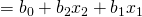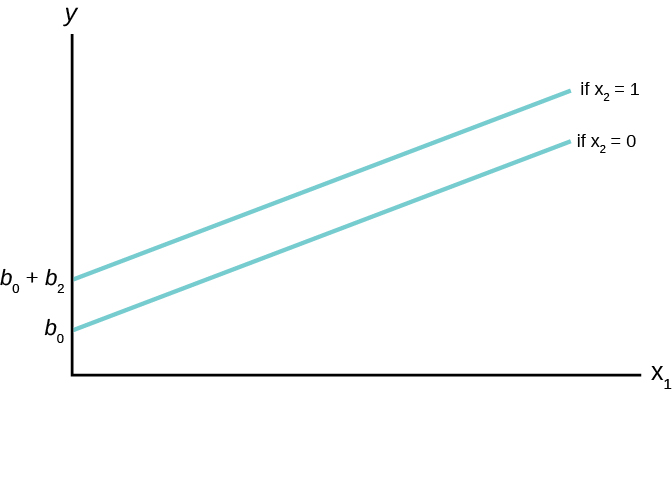where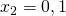. X2 is the dummy variable and X1 is some continuous random variable. The constant, b0, is the y-intercept, the value where the line crosses the y-axis. When the value of X2 = 0, the estimated line crosses at b0. When the value of X2 = 1 then the estimated line crosses at b0 + b2. In effect the dummy variable causes the estimated line to shift either up or down by the size of the effect of the characteristic captured by the dummy variable. Note that this is a simple parallel shift and does not affect the impact of the other independent variable; X1.This variable is a continuous random variable and predicts different values of y at different values of X1 holding constant the condition of the dummy variable.

An example of the use of a dummy variable is the work estimating the impact of gender on salaries. There is a full body of literature on this topic and dummy variables are used extensively. For this example the salaries of elementary and secondary school teachers for a particular state is examined. Using a homogeneous job category, school teachers, and for a single state reduces many of the variations that naturally effect salaries such as differential physical risk, cost of living in a particular state, and other working conditions. The estimating equation in its simplest form specifies salary as a function of various teacher characteristic that economic theory would suggest could affect salary. These would include education level as a measure of potential productivity, age and/or experience to capture on-the-job training, again as a measure of productivity. Because the data are for school teachers employed in a public school districts rather than workers in a for-profit company, the school district’s average revenue per average daily student attendance is included as a measure of ability to pay. The results of the regression analysis using data on 24,916 school teachers are presented below.

Earnings Estimate for Elementary and Secondary School Teachers
Variable Regression Coefficients (b) Standard Errors of the estimates
for teacher’s earnings function (sb)
Intercept 4269.9
Gender (male = 1) 632.38 13.39
Total Years of Experience 52.32 1.10
Years of Experience in Current District 29.97 1.52
Education 629.33 13.16
Total Revenue per ADA 90.24 3.76.725
n 24,916

The coefficients for all the independent variables are significantly different from zero as indicated by the standard errors. Dividing the standard errors of each coefficient results in a t-value greater than 1.96 which is the required level for 95% significance. The binary variable, our dummy variable of interest in this analysis, is gender where male is given a value of 1 and female given a value of 0. The coefficient is significantly different from zero with a dramatic t-statistic of 47 standard deviations. We thus cannot accept the null hypothesis that the coefficient is equal to zero. Therefore we conclude that there is a premium paid male teachers of ?632 after holding constant experience, education and the wealth of the school district in which the teacher is employed. It is important to note that these data are from some time ago and the ?632 represents a six percent salary premium at that time. A graph of this example of dummy variables is presented below.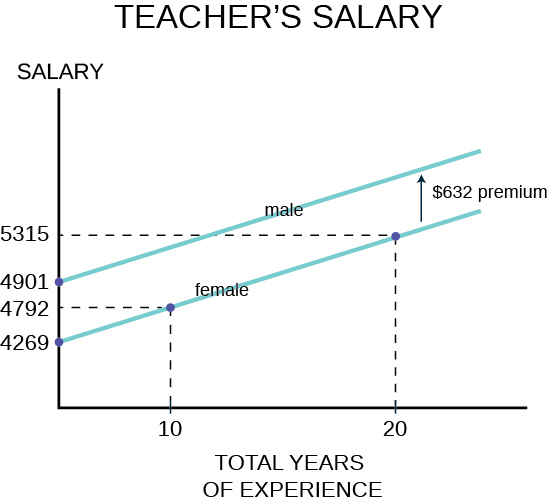In two dimensions, salary is the dependent variable on the vertical axis and total years of experience was chosen for the continuous independent variable on horizontal axis. Any of the other independent variables could have been chosen to illustrate the effect of the dummy variable. The relationship between total years of experience has a slope of ?52.32 per year of experience and the estimated line has an intercept of ?4,269 if the gender variable is equal to zero, for female. If the gender variable is equal to 1, for male, the coefficient for the gender variable is added to the intercept and thus the relationship between total years of experience and salary is shifted upward parallel as indicated on the graph. Also marked on the graph are various points for reference. A female school teacher with 10 years of experience receives a salary of ?4,792 on the basis of her experience only, but this is still ?109 less than a male teacher with zero years of experience.

A more complex interaction between a dummy variable and the dependent variable can also be estimated. It may be that the dummy variable has more than a simple shift effect on the dependent variable, but also interacts with one or more of the other continuous independent variables. While not tested in the example above, it could be hypothesized that the impact of gender on salary was not a one-time shift, but impacted the value of additional years of experience on salary also. That is, female school teacher’s salaries were discounted at the start, and further did not grow at the same rate from the effect of experience as for male school teachers. This would show up as a different slope for the relationship between total years of experience for males than for females. If this is so then females school teachers would not just start behind their male colleagues (as measured by the shift in the estimated regression line), but would fall further and further behind as time and experienced increased.

The graph below shows how this hypothesis can be tested with the use of dummy variables and an interaction variable.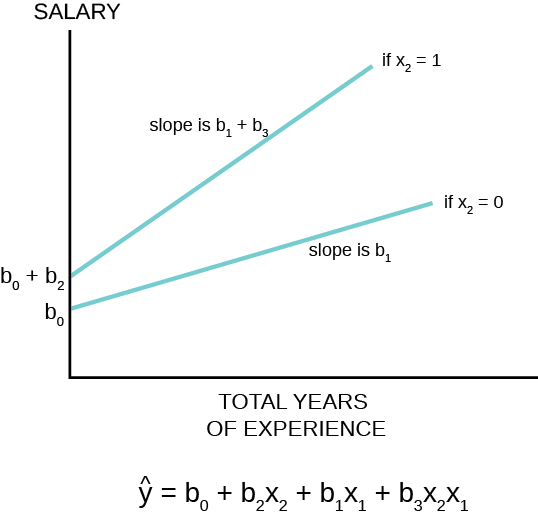The estimating equation shows how the slope of X1, the continuous random variable experience, contains two parts, b1 and b3. This occurs because of the new variable X2 X1, called the interaction variable, was created to allow for an effect on the slope of X1 from changes in X2, the binary dummy variable. Note that when the dummy variable, X2 = 0 the interaction variable has a value of 0, but when X2 = 1 the interaction variable has a value of X1. The coefficient b3 is an estimate of the difference in the coefficient of X1 when X2 = 1 compared to when X2 = 0. In the example of teacher’s salaries, if there is a premium paid to male teachers that affects the rate of increase in salaries from experience, then the rate at which male teachers’ salaries rises would be b1 + b3 and the rate at which female teachers’ salaries rise would be simply b1. This hypothesis can be tested with the hypothesis: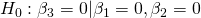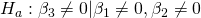This is a t-test using the test statistic for the parameter β3. If we cannot accept the null hypothesis that β3=0 we conclude there is a difference between the rate of increase for the group for whom the value of the binary variable is set to 1, males in this example. This estimating equation can be combined with our earlier one that tested only a parallel shift in the estimated line. The earnings/experience functions in (Figure) are drawn for this case with a shift in the earnings function and a difference in the slope of the function with respect to total years of experience.

A random sample of 11 statistics students produced the following data, where x is the third exam score out of 80, and y is the final exam score out of 200. Can you predict the final exam score of a randomly selected student if you know the third exam score?

Table showing the scores on the final exam based on scores from the third exam.
x (third exam score) y (final exam score)
65 175
67 133
71 185
71 163
66 126
75 198
67 153
70 163
71 159
69 151
69 159
Scatter plot showing the scores on the final exam based on scores from the third exam.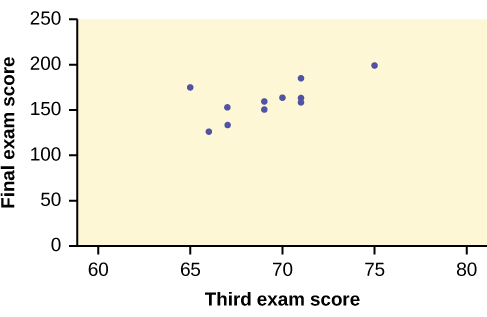Suppose that you have at your disposal the information below for each of 30 drivers. Propose a model (including a very brief indication of symbols used to represent independent variables) to explain how miles per gallon vary from driver to driver on the basis of the factors measured.

Information:
1. miles driven per day
2. weight of car
3. number of cylinders in car
4. average speed
5. miles per gallon
6. number of passengers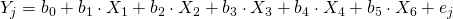Consider a sample least squares regression analysis between a dependent variable (Y) and an independent variable (X). A sample correlation coefficient of −1 (minus one) tells us that

1. there is no relationship between Y and X in the sample
2. there is no relationship between Y and X in the population
3. there is a perfect negative relationship between Y and X in the population
4. there is a perfect negative relationship between Y and X in the sample.

d. there is a perfect negative relationship between Y and X in the sample.

In correlational analysis, when the points scatter widely about the regression line, this means that the correlation is

1. negative.
2. low.
3. heterogeneous.
4. between two measures that are unreliable.

b. low

### Chapter Review

It is hoped that this discussion of regression analysis has demonstrated the tremendous potential value it has as a tool for testing models and helping to better understand the world around us. The regression model has its limitations, especially the requirement that the underlying relationship be approximately linear. To the extent that the true relationship is nonlinear it may be approximated with a linear relationship or nonlinear forms of transformations that can be estimated with linear techniques. Double logarithmic transformation of the data will provide an easy way to test this particular shape of the relationship. A reasonably good quadratic form (the shape of the total cost curve from Microeconomics Principles) can be generated by the equation: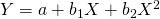where the values of X are simply squared and put into the equation as a separate variable.

There is much more in the way of econometric “tricks” that can bypass some of the more troublesome assumptions of the general regression model. This statistical technique is so valuable that further study would provide any student significant, statistically significant, dividends.

### Glossary

Residual or “error”
the value calculated from subtracting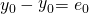. The absolute value of a residual measures the vertical distance between the actual value of y and the estimated value of y that appears on the best-fit line.
Sum of Squared Errors (SSE)
the calculated value from adding up all the squared residual terms. The hope is that this value is very small when creating a model.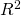– Coefficient of Determination
This is a number between 0 and 1 that represents the percentage variation of the dependent variable that can be explained by the variation in the independent variable. Sometimes calculated by the equation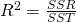where SSR is the “Sum of Squares Regression” and SST is the “Sum of Squares Total.” The appropriate coefficient of determination to be reported should always be adjusted for degrees of freedom first.